• Submissions# A Nano Capacitor Including Graphene Layers Composed with Doped Boron and Nitrogen

Seyedehmarzieh Sadatchoobeh1, Karim Zare1 and Majid Monajjemi2*

1Department of Chemistry, Islamic Azad University, Iran

2Department of Chemical Engineering, Islamic Azad University, Iran

*Corresponding author: Majid Monajjemi, Department of Chemical Engineering, Central Tehran Branch, Islamic Azad University, Tehran, Iran

Submission: December 18, 2017; Published: February 23, 2018

DOI: 10.31031/RDMS.2018.03.000573ISSN: 2576-8840
Volume3 Issue5

#### Abstract

In this study, it has been found a configuration including dopant atoms which are replaced in a diagonal line through hand-running atoms from each other. Those are more favorable energetically, and the configuration with atoms of boron and nitrogen are more stable with binding energies. This phenomenon confirms the homogeneous B-substitution that may be easier than the other atoms such as N-substitution. The root of these differences might be affected the structure of B-C bonds which are about 0.5% longer than the C-C bond. Band-gap is opened through the effect of substitution B atom doping on the structure of graphene and the Fermi levels lies in the valence and conduction bands. It has been shown a model of a nanoscale dielectric capacitor composed of a few dopants including metallic graphene layers separated by an insulating medium containing a few h-BN layers

Keywords: Nano capacitor; Graphene; Doping; Boron nitride sheet

#### Introduction

Graphene individually has a structural flexibility due to its electronic properties. Because of electronic flexibilities, graphene might be fitted chemically in a deposit metal atoms on top , The deposit molecules on it , incorporated nitrogen and boron in their structures  and doped atoms inside the sheets. They can be used in a field of the electronics, capacitors, superconductors, in batteries and diodes. The considerable tends of graphene are the Dirac solid, with the electron energies being linearly dependent on the wave vectors near the vertices of the hexagonal Brillouin zones  . Complete histories for the rising of graphene have given by Geim & Novoselov .

In this work the properties of various dopants with the electronic structures of a few-layer graphene into two side electrodes in the capacitor has been studied. In this matter, it has been investigated a nano-capacitor through B- and N-doped graphene bilayers employing different strategy for its structure and properties. The B and N atoms are the natural templates for doping in graphene due to their similar atomic size as that of C and of their hole acceptors and electron donors characters for substitution Band N-doping, respectively.

It has been modeled by two configurations of doped bi-layer graphene including various atoms such as (h-BN)n sheets (n=1- 4). Our nanoscale capacitors model are composed of hexagonal (h-BN)n layers (n=1- 4), which are stacked between two graphene electrodes. The single and multi-layers of (h-BN)n are wide band gap insulators, so they can be applied as a dielectric material between metallic graphene layers. An effort has been accomplished for understanding the theoretical basis for the nanoscale dielectric capacitors which are professional to another system of energy storage. In fact, capacitor at nanoscale has been developed as one of the most expecting energies storages mediums [6,7].

That h-BN layers of each thickness might be grown on the graphene electrodes it has been already shown both experimentally  and theoretically [9,10]. It is also perpendicular carbon to grow BN chains (also available) on top of the graphene and h-BN single layers [11,12].

At the nanoscales, since the separation thickness of capacitors model can be as smaller as a nanometer size, the stored energy has to be calculated from the first principle calculation [12-14] which allows to treat the distribution of only one kind of excess positive or negative charges at a time (in the same system) [13-16]. In this work it has found a significant increasing of capacitance and negative quantum capacitance based on percentages of B dopants and thickness of h-BN layers.

Via applying an external electric fields perpendicular to the graphene electrodes the charge separation might be achieved in this situation and simulates of the operation by the surface charge with opposite sign initially stored on different doped plates create perpendicular electric fields. The shorting of these two plates and hence the discharges of the capacitors are prevented by placing sufficient quantities of h-BN layers between doped graphene plates.

Based on electrostatic theory the geometric capacitance densities, "cg", is related to the applied Voltage,ΔVapp as the equation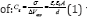where σ is the surface charge densities, εr, ε0 are the dielectric constant and absolute permittivities respectively. Ajayan  has found a significant increasing in the capacitance below the thickness of "5nm", more than what is predicted by classical electrostatic. This unusual increase in a capacitance is because of the negative quantum capacitance that this particular materials system exhibits (Figure 1).

Figure 1: Nano-capacitors models.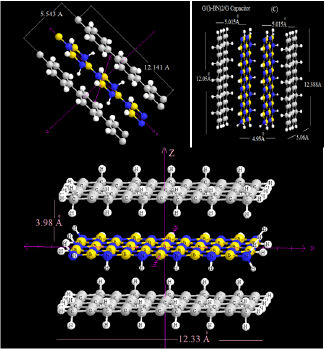#### Theoretical Back Grounds and Model

Figure 2: Density versus atomic position.The patterns of negative quantum effects in the interaction between the gold electrodes and h-BN films have investigated by Ajayan . Ajayan have determined the dielectric permittivities as a function of dielectric size through ab-inito calculation using the SIESTA atomistic simulation package .The Nano-dielectric- capacitor models or NDC have been successfully used for simulating stacking structure of G/h-BN/G . Density functional theory with the van der Waals density functional for modeling the exchange- correlation energy of Au and h-BN have been employed through a relativistic pseudo-potential method [19-21] (Figure 2).

It has been considered two isolated metal-doped-graphene (MDG) layers where each one is fully charged by Q electrons per primitive unit-cells . The NDC models with (h-BN)n layers (n=1, 2, 3) as dielectric are covered through two parallel MDG (M=Mg, Al, Si, B) layers. In our model the interlayer distances for h-BN varies in the range of {3.0-5.0} A, which they are the beset interaction, while the 3.55 is in a reasonable data and agreement with the experimental variables (3.306 A)  and also theoretical values  (Figure 3).

Figure 3: LOL color field map.Recently a few works [7,16,22-25] indicate an abnormal increasing in the capacitance along decreasing size of nano-system including negative quantum capacitance. These effects arise from many-body interaction because the chemical potentials of the electron decrease with increasing of electron density. Maccuci [26,27] exhibited a capacitance of quantum dots. Another work on non-linear quantum capacitances  and atomic junctions  has been investigated by Wang et al. The hybrid capacitances and then quantum capacitances are related to the net capacitances, C, with the relationand the values of CQ are many orders of magnitude greater than the Cg, and hence its effect usually appears only in the very small systems .

The QM components are development of the density of state (DoS) for the metal electrodes, and their Thomas-Fermi screening length. Hence the hybrid capacitances of each nano-capacitors architecture are given as: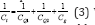Where C_t is the total capacitance of the nano-capacitors, in addition Cg is the classical capacitances, CQ1 and CQ2 are the quantum capacitance due to their finite DoS of the metal doped graphene electrodes (M1G, and M2G, respectively).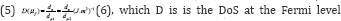[30,31] of either electrodes.which for metal for metal=Au . Hence the hybrid capacitances due to its composition of both classical and quantum capacitances can be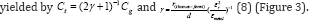#### Computational Details

Calculations were performed by GAMESS-US package . In this work, we have focused on getting the results from DFT methods for the non-bonded interaction of graphene sheets composed with boron and nitrogen doping. They also are monotonous through the comparison between different situations. Density functional theory with the van der Waals density functional (vdW-DF) has been applied to model the exchange-correlation energy of doped graphene and h-BN . The double Z-basis sets with polarization orbital (DZP) was used for doping while single Z-basis sets including polarization orbitals (SZP) was employed for the h-BN layers, respectively. The m062x, m06-L, and m06-HF are new method within good correspondence in the non-bonded calculation and are suitable for the energy of the distances between two fragments in the capacitors. For a non-covalent interaction; B3LYP is unable for describing van der Waals [33,34] capacitor systems in mediumrange interactions, such as the interactions of two electrode and dielectric sheets.

In addition, some recent study has shown the inaccuracy of the medium-range exchange energies leads to large systematic error in the prediction of molecular properties [35-39]. Graphene is known in 2-D honeycomb structures and the B and N doped graphene will be assumed to have a similar structure, unless violated by energies minimization considerations. A monolayer of G was optimized and allowed for relax to its minimum energies structure; it contains 76 atoms with zigzag edges. The edges were saturated with the hydrogen atoms to neutralize the valance of terminal carbons, reducing edges effects .

Geometry optimizations and electronic structure calculation have been performed using the m06 (DFT) which the approaches are based on an iterative solution of the Kohn-Sham equation  of the density function theory in a plane-wave set with the projector-augmented wave pseudo-potentials. The Perdew-Burke- Ernzerhof (PBE)  exchange-correlation (XC) functional of the generalized gradient approximation (GGA) is adopted. The optimizations of the lattice constants and the atomic coordinates are made by the minimization of the total energies. The dimension of capacitors has been set as 12.388x3. 95x8.52 Å (scheme 1) and the sheets are separated by various distance (Table 1 & 2) along the perpendicular direction to avoid interlayer interaction. During all of the calculations processes, except for the band determination, the partial occupancy was treated using the tetrahedron methodology with Blochl  corrections.

In our model the electrodes have doped through various percentage of boron atoms which are likely to adjust for surrounding C atoms of the host. Therefore when the graphene sheet is doped with one boron atoms, the boron atoms also undergoes sp2 hybridization and due to the nearly same size of C and B, no significant distortion in 2-D structure of graphene are expected, except for change in adjoining bond lengths. By doping boron atoms in graphene Fermi level shifts significantly below the Dirac point resulting into a p-type doping and the symmetries of graphene have broken in two graphene sub-lattices because of introduction of B atom and causes for changing the behavior of graphene from semimetal to conductors. The charges transfer and electrostatic potential-derived charges were also calculated using the Besler , Chirlian , Brneman  (Figure 4).

Figure 4: Contour map of ELF.Figure 5: Average local ionization energy.The charges calculation method based on molecular electrostatic potentials (MESP) fitting are not well-suited for treating larger system where some of the innermost atoms are located far away from the points at which the MESP is computed. In such a condition, variation of the innermost atomic charge will not lead to significant changing of the MESP outside of the molecule, meaning that the accurate value for the innermost atomic charge are not well-determined by MESP outside of the molecules. The representative atomic charges for molecule should be computed as the average value over several molecular conformations. A detailed overview of the effect of the basis set and the Hamiltonian on the charge distribution can be found in [46,47]. We have also extracted the charges density profiles from the first-principles calculation through an averaging process described [48,49].

Although infinite graphene sheet are intrinsically metallic, our BG system exhibits an increasing in the metallic properties. The interaction energies for capacitor were calculated in all items as the equation: + ΔES(eV) = {Ec -(2ECopant-G + Eh-BN)}+EBSSE (9) where the "ΔEs " is the stability energy of capacitor. The fundamental of this work is based on our previous works [50-84].

#### Results and Discussion

In this work, the values of the distance between graphene layers capping h-BN layers, dielectric constants of the layered h-BN sheets (k), magnitude of the charge on the graphene electrode, the stabilities energies of capacitors (in eV) and potential difference between the electrodes of graphene plates are listed in Tables 1-3. Using various percentages dopants in tables indicate proper situations for boron and nitrogen dopants in graphene electrodes.

Although the dielectric strength can be deduced from the band structure of BN spacer, here we have calculated the dielectric constant straightly from (eq.1), which is much more accurate than the other methods. The potential energy difference between the two graphene layers,are shown in Table 3. In addition, according to electronic structures we have considered two isolated graphene layers, which each one is doped by boron atoms in various distances. They have been charged by q+m-G and q-m-G electrons per primitive unit-cellswhich are shown in Tables 1-3.

The atomic structures, interlayer spacing, relative positions of the layers and the cell parameters are optimized. It is obvious that for BN spacer consisting of a few layers, the different voltages among electrodes depend on various conditions such as nonequilibrium state, the coupling between graphene and adjacent h-BN, etc. and require a thorough analysis. In Tables 1-3, we have shown comparison among the stabilityand Gap Energy (eV) of various dopants' percentage.

#### Conclusion

In this study, we have shown the model of a nanoscale dielectric capacitor composed of various percentage dopants including h-BN and graphene sheets separated by an insulating medium containing a few layers. We showed that in this model, the percentage dopants play significant roles in the nano capacitors. The capacitor with medium percentage of boron nitrides dopants has the high dielectric constant. Our theoretical results show that the relative permittivity of dielectric can be higher than that of no percentage of boron nitride.

We at Crimson Publishing are a group of people with a combined passion for science and research, who wants to bring to the world a unified platform where all scientific know-how is available read more...